CAT  >  Test: Data Tabulation & Caselets - 2

# Test: Data Tabulation & Caselets - 2

Test Description

## 15 Questions MCQ Test Topic-wise Past Year Questions for CAT | Test: Data Tabulation & Caselets - 2

Test: Data Tabulation & Caselets - 2 for CAT 2023 is part of Topic-wise Past Year Questions for CAT preparation. The Test: Data Tabulation & Caselets - 2 questions and answers have been prepared according to the CAT exam syllabus.The Test: Data Tabulation & Caselets - 2 MCQs are made for CAT 2023 Exam. Find important definitions, questions, notes, meanings, examples, exercises, MCQs and online tests for Test: Data Tabulation & Caselets - 2 below.
Solutions of Test: Data Tabulation & Caselets - 2 questions in English are available as part of our Topic-wise Past Year Questions for CAT for CAT & Test: Data Tabulation & Caselets - 2 solutions in Hindi for Topic-wise Past Year Questions for CAT course. Download more important topics, notes, lectures and mock test series for CAT Exam by signing up for free. Attempt Test: Data Tabulation & Caselets - 2 | 15 questions in 25 minutes | Mock test for CAT preparation | Free important questions MCQ to study Topic-wise Past Year Questions for CAT for CAT Exam | Download free PDF with solutions
 1 Crore+ students have signed up on EduRev. Have you?
Test: Data Tabulation & Caselets - 2 - Question 1

### Directions for Questions: Answer the questions on the basis of the information given below.  The total electricity production of five thermal power plants in India in year 2009-10 is given in the table below. Capacity utilization for any power plant is the percentage of maximum capacity, of that power plant, which is used for power production. Maximum capacity (100%) = Capacity utilization (In %) + Unutilized production (In %) (2015)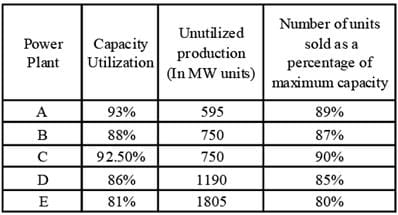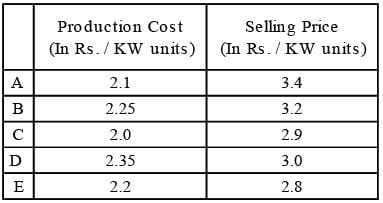Total cost of production = Units Produced (in KW) × Production Cost (in Rs. / KW units) Total Revenue = Units Sold (in KW) × Selling price (in Rs. / KW units) Profitability = (Revenue - Cost / Cost) × 100 Q. Which of the following represents the decreasing order of units sold by the given 5 power plants?

Detailed Solution for Test: Data Tabulation & Caselets - 2 - Question 1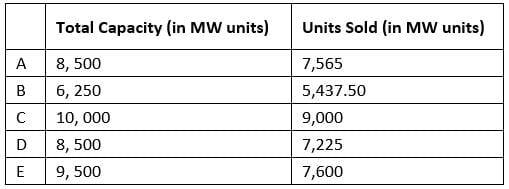According to above table, The correct order is C > E > A > D > B

Test: Data Tabulation & Caselets - 2 - Question 2

### Directions for Questions: Answer the questions on the basis of the information given below.  The total electricity production of five thermal power plants in India in year 2009-10 is given in the table below. Capacity utilization for any power plant is the percentage of maximum capacity, of that power plant, which is used for power production. Maximum capacity (100%) = Capacity utilization (In %) + Unutilized production (In %) (2015)Total cost of production = Units Produced (in KW) × Production Cost (in Rs. / KW units) Total Revenue = Units Sold (in KW) × Selling price (in Rs. / KW units) Profitability = (Revenue - Cost / Cost) × 100 Q. Which of the following statements is true?

Detailed Solution for Test: Data Tabulation & Caselets - 2 - Question 2Only statement 'b' is true.

Test: Data Tabulation & Caselets - 2 - Question 3

### Directions for Questions: Answer the questions on the basis of the information given below.  The total electricity production of five thermal power plants in India in year 2009-10 is given in the table below. Capacity utilization for any power plant is the percentage of maximum capacity, of that power plant, which is used for power production. Maximum capacity (100%) = Capacity utilization (In %) + Unutilized production (In %) (2015)Total cost of production = Units Produced (in KW) × Production Cost (in Rs. / KW units) Total Revenue = Units Sold (in KW) × Selling price (in Rs. / KW units) Profitability = (Revenue - Cost / Cost) × 100 Q. In the given year, if capacity of power plant B had 12.5% of the total power capacity of India, and thermal power capacity of India is 95% of its total power capacity. The total capacity of these 5 thermal power plants was what percentage of the total thermal power capacity of India?

Detailed Solution for Test: Data Tabulation & Caselets - 2 - Question 3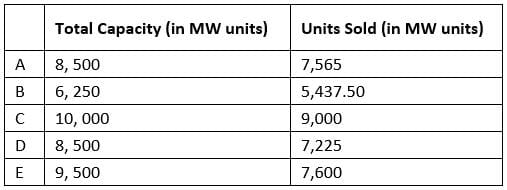∵ Total capacity of power plant B of India = 6250.
∴ Total capacity of India = 6,250 × 100/12.5
= 50,000 MW units
∴ Thermal capacity of India = 95% of total capacity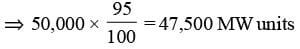Total capacity of these five power plants = (8500 + 6250 + 10,000 + 8500 + 9500) = 42,750 MW units
300 + 3400 = 3700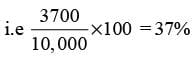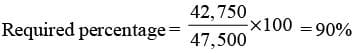Test: Data Tabulation & Caselets - 2 - Question 4

Directions for Questions: Answer the questions on the basis of the information given below.
The total electricity production of five thermal power plants in India in year 2009-10 is given in the table below. Capacity utilization for any power plant is the percentage of maximum capacity, of that power plant, which is used for power production.
Maximum capacity (100%) = Capacity utilization (In %) + Unutilized production (In %)

(2015)Total cost of production = Units Produced (in KW) × Production Cost (in Rs. / KW units)
Total Revenue = Units Sold (in KW) × Selling price (in Rs. / KW units)
Profitability = (Revenue - Cost / Cost) × 100

Q. Which power plant had the third highest profitability?

Detailed Solution for Test: Data Tabulation & Caselets - 2 - Question 4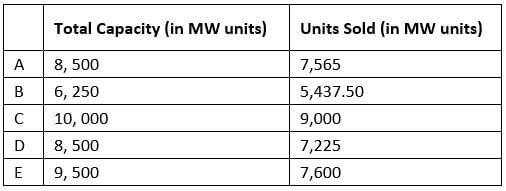Profitability can be compared by comparing the ratio of total revenue to total cost.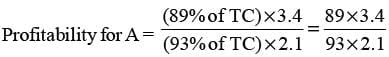= 1.549.
(where TC is the total capacity of that power plant.)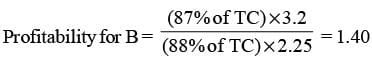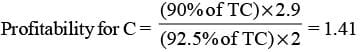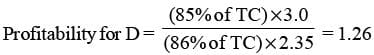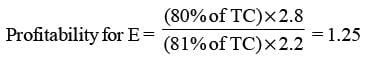So, B has the third highest profitability.

Test: Data Tabulation & Caselets - 2 - Question 5

Directions for Questions: Answer the questions on the basis of the information given below.
The table given below shows the data related to a few key financial indicators for fourteen European countries in the FY 2011-12.

(2013)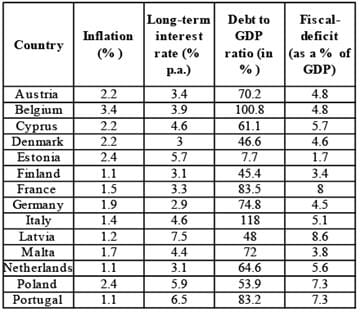Q. If the Fiscal-deficit of France was x Euros, which was 50% more than that of Belgium, then what was the Debt (in Euros) of Belgium in FY 2011-12?

Detailed Solution for Test: Data Tabulation & Caselets - 2 - Question 5

Let the GDP of Belgium by E Euros.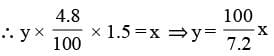Hence, the debt (in Euros) of Belgium) in FY 2011–12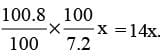Test: Data Tabulation & Caselets - 2 - Question 6

Directions for Questions: Answer the questions on the basis of the information given below.
The table given below shows the data related to a few key financial indicators for fourteen European countries in the FY 2011-12.

(2013)Q. The countries with the Long-term interest rate less than 4% per annum, Debt to GDP ratio less than 60% and Fiscal-deficit not more than 4.6% were given a AAA rating. The number of countries rated AAA among the fourteen in FY 2011-12 was

Detailed Solution for Test: Data Tabulation & Caselets - 2 - Question 6

According to given data Two countries, viz. Denmark and Finalnd, were rated AAA among the fourteen in FY 2011–12.

Test: Data Tabulation & Caselets - 2 - Question 7

Directions for Questions: Answer the questions on the basis of the information given below.
The table given below shows the data related to a few key financial indicators for fourteen European countries in the FY 2011-12.

(2013)Q. If the GDP (in Euros) of Finland was 50% more than that of Italy, then by what percent was the Fiscaldeficit (in Euros) of Italy more/less than that of Finland in FY 2011-12?

Detailed Solution for Test: Data Tabulation & Caselets - 2 - Question 7

Let the GDP (in Euros) of Italy = x.
Therefore. the GDP (in Euros) of Finland = 1.5x.
The fiscal–deficit of.
Italy = (x + 5.1 / 100) = 0.051 x
Finland = (1.5x x 3.4 / 100) = 0.034 x 1.5x = 0.051 x.
Hence, the required percentage = 0.

Test: Data Tabulation & Caselets - 2 - Question 8

Directions for Questions: Seven companies A, B, C, D, E, F and G are engaged in production of two items I and II. The comparative data about production of these items by the seven companies is given in the following Graph and Table. Study them carefully to answer the questions that follow.

(2011)

PERCENTAGE OF THE TOTAL PRODUCTION PRODUCED BY THE SEVEN COMPANIES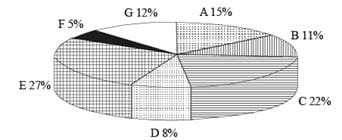Cost of the total production (both items together) by seven companies = ₹ 25 crores
RATIO OF PRODUCTION BETWEEN ITEMS I AND II AND THE PERCENT PROFIT EARNED FOR THE TWO ITEMS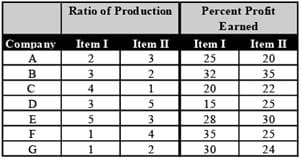Q. Cost of production of item I by company F is what percent of the cost of production of item II by company D?

Detailed Solution for Test: Data Tabulation & Caselets - 2 - Question 8

According to the question, cost of total production by all the seven companies = ₹ 25 crores.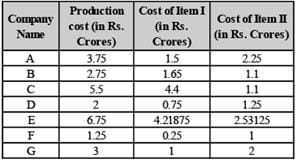Required % age = 0.25 / 1.25 x 100 = 20%

Test: Data Tabulation & Caselets - 2 - Question 9

Directions for Questions: Seven companies A, B, C, D, E, F and G are engaged in production of two items I and II. The comparative data about production of these items by the seven companies is given in the following Graph and Table. Study them carefully to answer the questions that follow.

(2011)

PERCENTAGE OF THE TOTAL PRODUCTION PRODUCED BY THE SEVEN COMPANIESCost of the total production (both items together) by seven companies = ₹ 25 crores
RATIO OF PRODUCTION BETWEEN ITEMS I AND II AND THE PERCENT PROFIT EARNED FOR THE TWO ITEMSQ. What is the total profit earned by company G for items I and II together?

Detailed Solution for Test: Data Tabulation & Caselets - 2 - Question 9

According to the question, cost of total production by all the seven companies = ₹ 25 crores.Total Profit earned by G for both items I and II = 30% of 1 crore  + 24% of 2 crore = 30 lakhs + 48 lakhs = 78 lakhs.

Test: Data Tabulation & Caselets - 2 - Question 10

Directions for Questions: Seven companies A, B, C, D, E, F and G are engaged in production of two items I and II. The comparative data about production of these items by the seven companies is given in the following Graph and Table. Study them carefully to answer the questions that follow.

(2011)

PERCENTAGE OF THE TOTAL PRODUCTION PRODUCED BY THE SEVEN COMPANIESCost of the total production (both items together) by seven companies = ₹ 25 crores
RATIO OF PRODUCTION BETWEEN ITEMS I AND II AND THE PERCENT PROFIT EARNED FOR THE TWO ITEMSQ. What is the ratio of the cost of production of item I by company A to the cost of production of item I by company D?

Detailed Solution for Test: Data Tabulation & Caselets - 2 - Question 10

According to the question, cost of total production by all the seven companies = ₹ 25 crores.Cost of production of I by company A / Cost of production of I by company D
= 1.5 / 0.75 = 2 / 1 = 2 : 1

Test: Data Tabulation & Caselets - 2 - Question 11

Directions for Questions: Seven companies A, B, C, D, E, F and G are engaged in production of two items I and II. The comparative data about production of these items by the seven companies is given in the following Graph and Table. Study them carefully to answer the questions that follow.

(2011)

PERCENTAGE OF THE TOTAL PRODUCTION PRODUCED BY THE SEVEN COMPANIESCost of the total production (both items together) by seven companies = ₹ 25 crores
RATIO OF PRODUCTION BETWEEN ITEMS I AND II AND THE PERCENT PROFIT EARNED FOR THE TWO ITEMSQ. The cost of production of both items together by company E is equal to the total cost of production of both items together by which of the two companies?

Detailed Solution for Test: Data Tabulation & Caselets - 2 - Question 11

According to the question, cost of total production by all the seven companies = ₹ 25 crores.Cost of production of both items together by company E = 6.75 crores Cost of production of C & D = 7.50 crores; of B & G = 5.75 crores; of A and D = 5.75 crores; of C & F = 6.75 crores.

Test: Data Tabulation & Caselets - 2 - Question 12

Directions for Questions: Seven companies A, B, C, D, E, F and G are engaged in production of two items I and II. The comparative data about production of these items by the seven companies is given in the following Graph and Table. Study them carefully to answer the questions that follow.

(2011)

PERCENTAGE OF THE TOTAL PRODUCTION PRODUCED BY THE SEVEN COMPANIESCost of the total production (both items together) by seven companies = ₹ 25 crores
RATIO OF PRODUCTION BETWEEN ITEMS I AND II AND THE PERCENT PROFIT EARNED FOR THE TWO ITEMSQ. What is the total of the cost of production of item I by company A and the cost of production of item II by company B?

Detailed Solution for Test: Data Tabulation & Caselets - 2 - Question 12

According to the question, cost of total production by all the seven companies = ₹ 25 crores.Cost of production of item I by A and item II by B = 1.5 + 1.10 = 2.60 crores.

Test: Data Tabulation & Caselets - 2 - Question 13

Directions for Questions: Read the table carefully and answer the questions that follow:-Given below are data points on the India economy from 2005 - 2010:

(2010)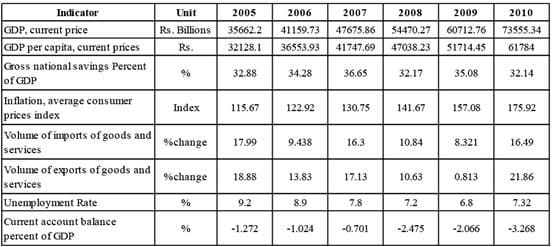*Per capita GDP is arrived by dividing GDP by population.

Q. What is the ratio of the current account balance in 2010 to current account balance 2005?

Detailed Solution for Test: Data Tabulation & Caselets - 2 - Question 13

Current account balance in 2010
= – 3.268% of 73555.34
= – 2403.78 (in ₹ billions)
Current account balance in 2005
= – 1.272% of 35662.2
= – 453.62 (in ₹ billions)
∴ Ratio = – 2403.78 / - 453.62 = 5.36 (approx.)

Test: Data Tabulation & Caselets - 2 - Question 14

Directions for Questions: Read the table carefully and answer the questions that follow:-Given below are data points on the India economy from 2005 - 2010:

(2010)*Per capita GDP is arrived by dividing GDP by population.

Q. Read the statements given below:
1. Exports were more than imports in 2006
2. Imports were more than exports in 2009
3. Export increased at faster rate than imports during period 2005 to 2010
Which of the statements is necessarily true?

Test: Data Tabulation & Caselets - 2 - Question 15

Directions for Questions: Read the table carefully and answer the questions that follow:-Given below are data points on the India economy from 2005 - 2010:

(2010)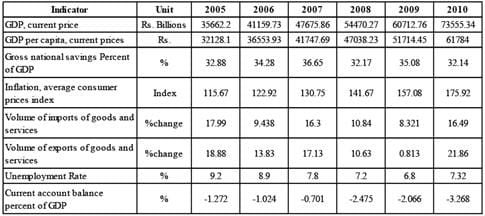*Per capita GDP is arrived by dividing GDP by population.

Q. What was the approximate number of unemployed persons in 2006

Detailed Solution for Test: Data Tabulation & Caselets - 2 - Question 15

The number of unemployed persons
= unemployment rate × population
= Unemployment rate × GDP / GDP percapita
= 8.9 / 100 x 41159.73 / 36553.93
= 0.1002 Billion ≈ 100 million.

## Topic-wise Past Year Questions for CAT

43 docs|31 tests
 Use Code STAYHOME200 and get INR 200 additional OFF Use Coupon Code
Information about Test: Data Tabulation & Caselets - 2 Page
In this test you can find the Exam questions for Test: Data Tabulation & Caselets - 2 solved & explained in the simplest way possible. Besides giving Questions and answers for Test: Data Tabulation & Caselets - 2, EduRev gives you an ample number of Online tests for practice

## Topic-wise Past Year Questions for CAT

43 docs|31 tests

### How to Prepare for CAT

Read our guide to prepare for CAT which is created by Toppers & the best Teachers